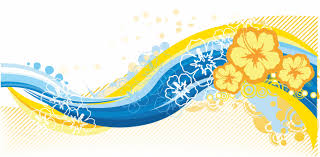# 멀티미디어 시스템 – Chapter 4. Vector Graphics and 3D Modeling

This chapter covers Vector graphics.## 1. Vector Graphic Characteristics

1. ### Vector Graphic

1. Main Characteristics
1. Compact (less memory than image for storage)
2. Scalable (No quality change with scaling, zoom in/out) → resolution-independent
2. Small file size and memory < bitmap image
1. Attractive for networked multimedia, but not widely used on WWW
2. SVG (W3C standard) < Shockwave Flash (SWF) (de facto standard)
3. Common formats for PDF, SVF, SWF
2. ### Modeling - Rendering

1. Modeling
1. Represent lines, curves, shapes,... by parameters of their
defining equations
2. Ex) line y = mx + c, store m and c (or endpoints)
2. Rendering
1. Compute pixels (location, color) which must be set in order to draw the line, curves,…
2. Generally, rendering result will exhibit 'Jaggies‘
1. Pixels' coordinates are integers, equations in vector graphic uses real numbers – no exact matching

## 2. Anti-aliasing

1. Example2. ### Anti-aliasing

1. Mitigate aliasing by coloring pixels in shades of grey (for a black line on figure in next page)
3. ### Rendering a vector object to produce an image made up of pixels

1. Jaggies - called aliasing
2. Ex) Line - continuous line must be approximated by discrete pixels of finite size
4. ### Primitive Shapes for Graphic

1. Generally, drawing programs such as Visio, Adobe illustrator, provide primitive shapes that can be easily represented mathematically
1. Rectangles and squares (may rounded corners)
2. Ellipses and circles
3. Straight lines, polylines and polygons
4. Smooth (Bézier) curves## 3. Open GL

1. ### OpenGL Geometric Primitives

1. Open Graphic Library by Silicon Graphic in 1992
1. 2D and 3D graphic standard API
2. All geometric primitives are specified by vertices2. ### Smooth (Bezier) Curves

1. Smooth curves completely specified by four control points
1. 2 endpoints (P0, P3)
2. 2 direction points (P1, P2)
2. Making a curve
1. Define a curve by length and direction of lines from endpoints to direction points3. ### Definition of Path

1. Define Bézier curves in terms of path
1. Closed path (curves connected end-to-end) and open path
2. Use Pen tool in drawing programs to build up path
4. ### Stroke and Fill

1. Apply Stroke to make path visible1. Like tracing with ink, specify width and color
2. Apply Fill to closed path or shape
1. Like coloring it in, specify color or a gradient fill or pattern fill3. Stroke Styles5. OpenGL Example - Gradient Fill6. Fill : Coloring inside path
Which is inside and outside?

1. Non-zero winding number rule5. ### Vector Transformations

1. Typical transformation of vector object can be done by changing stored vector values
1. Translation (linear movement)
2. Scaling (Zoom in and out)
3. Reflection
4. Rotation
5. Shearing (skewing)
2. Transformations## 4. 3D Vector Graphic – Modeling and Rendering

1. ### 3D Vector Graphics

1. Conceptually simple extension of 2-D
1. Add z-axis at right angles to x- and y-axes
2. Point is defined by (x, y, z) coordinates
3. Generalize coordinate geometry from 2D to 3D
2. Practically complicated and difficult
1. Requires 3D visualization skills
2. Complex tools, need high computational power
3. 3D modeling - rendering
1. Model - geometrical features of object
2. Rendering - surface characteristics, colors and textures
2. ### 3D Modeling

1. Three general approach to model 3D objects
1. Constructive solid geometry
1. Use of few geometric basis model such as cube, cylinder, sphere, pyramid
→ Set operations of union, intersection, difference
2. Free-form modeling
1. Use representation of an object’s boundary surface as the basis of model – ex) polygon mesh
3. Procedural modeling
1. Use of some algorithm or procedure
2. Ex) Fractal, Metaballs, Particle systems
2. Constructive solid geometry model3. Free-form modeling (Polygon Mesh)
1. represent dolphin' s boundary surface as polygon mesh4. Fractals (Procedural modeling)
1. In 1975, Benoit Mandelbrot
2. Self-similarity
1. Exhibit same structure at all levels of detail similar to whole structure ( each part is reduced size copy of whole)
2. Recursively generated5. Mandelbrot set3. ### 3D Rendering

1. A procedure giving a 3D realism to the model
1. Surface characteristics (shading), colors, textures, etc.
1. Means surface characteristics of object under different
lighting conditions or light sources such as – spots,
diffuse, ...
2. Need a computing model based on physics of light1. Shading - different coloring for each face of box or
2. giving a different amount of light for each face of box
1. Ray casting
1. Different color across each polygon mesh surface to simulate 3D object
2. Not considering light interaction between object2. Ray tracing
1. Take account of interaction between objects